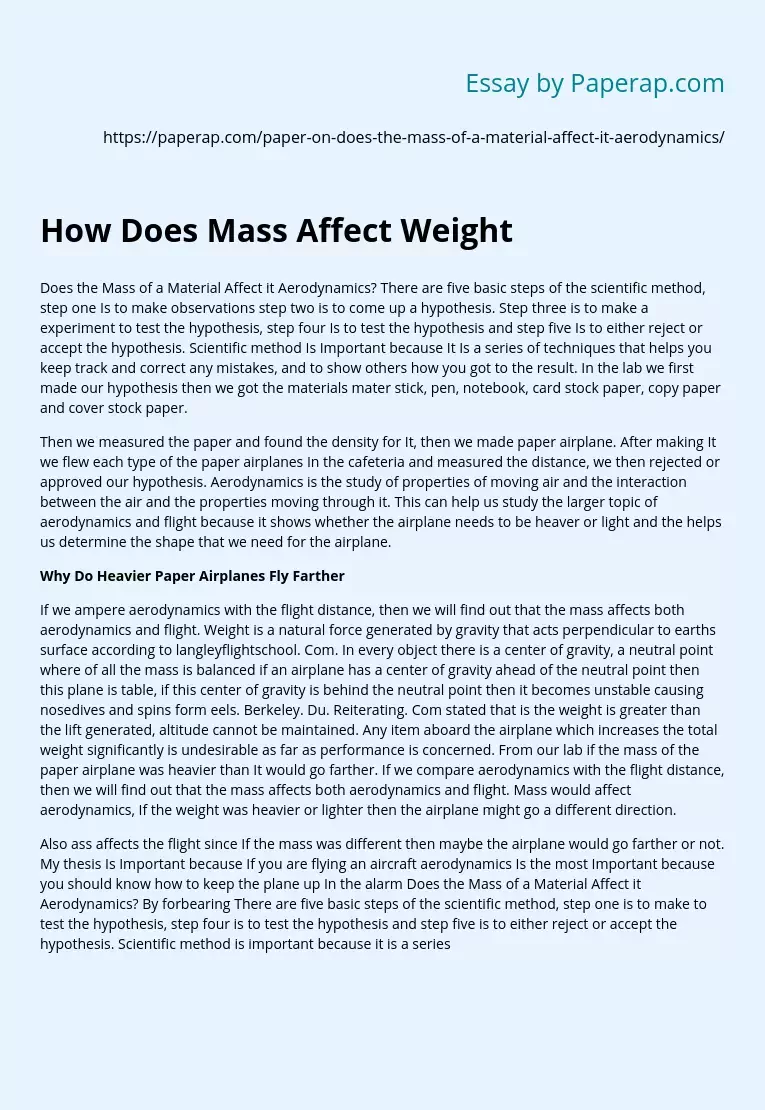# How Does Mass Affect Weight

Does the Mass of a Material Affect it Aerodynamics? There are five basic steps of the scientific method, step one Is to make observations step two is to come up a hypothesis. Step three is to make a experiment to test the hypothesis, step four Is to test the hypothesis and step five Is to either reject or accept the hypothesis. Scientific method Is Important because It Is a series of techniques that helps you keep track and correct any mistakes, and to show others how you got to the result.

In the lab we first made our hypothesis then we got the materials mater stick, pen, notebook, card stock paper, copy paper and cover stock paper.

Then we measured the paper and found the density for It, then we made paper airplane. After making It we flew each type of the paper airplanes In the cafeteria and measured the distance, we then rejected or approved our hypothesis. Aerodynamics is the study of properties of moving air and the interaction between the air and the properties moving through it.

This can help us study the larger topic of aerodynamics and flight because it shows whether the airplane needs to be heaver or light and the helps us determine the shape that we need for the airplane.

## Why Do Heavier Paper Airplanes Fly Farther

If we ampere aerodynamics with the flight distance, then we will find out that the mass affects both aerodynamics and flight. Weight is a natural force generated by gravity that acts perpendicular to earths surface according to langleyflightschool.

Get quality help nowRhizManVerified

Proficient in: Airplane4.9 (247)

“ Rhizman is absolutely amazing at what he does . I highly recommend him if you need an assignment done ”+84 relevant experts are online

Com. In every object there is a center of gravity, a neutral point where of all the mass is balanced if an airplane has a center of gravity ahead of the neutral point then this plane is table, if this center of gravity is behind the neutral point then it becomes unstable causing nosedives and spins form eels. Berkeley. Du. Reiterating. Com stated that is the weight is greater than the lift generated, altitude cannot be maintained. Any item aboard the airplane which increases the total weight significantly is undesirable as far as performance is concerned. From our lab if the mass of the paper airplane was heavier than It would go farther. If we compare aerodynamics with the flight distance, then we will find out that the mass affects both aerodynamics and flight. Mass would affect aerodynamics, If the weight was heavier or lighter then the airplane might go a different direction.

Also ass affects the flight since If the mass was different then maybe the airplane would go farther or not. My thesis Is Important because If you are flying an aircraft aerodynamics Is the most Important because you should know how to keep the plane up In the alarm Does the Mass of a Material Affect it Aerodynamics? By forbearing There are five basic steps of the scientific method, step one is to make to test the hypothesis, step four is to test the hypothesis and step five is to either reject or accept the hypothesis. Scientific method is important because it is a series paper.

Then we measured the paper and found the density for it, then we made paper airplane. After making it we flew each type of the paper airplanes in the concerned. From our lab if the mass of the paper airplane was heavier than it would mass affects both aerodynamics and flight. Mass would affect aerodynamics, if the mass affects the flight since if the mass was different then maybe the airplane would go farther or not. My thesis is important because if you are flying an aircraft aerodynamics is the most important because you should know how to keep the plane up in the air